Angular Momentum Formula

Angular momentum relates to how much an object is rotating. An object has a constant angular momentum when it is neither speeding up nor slowing down. It is equal to the cross product of a length and a linear momentum. The length is between a center of rotation and a point where the linear momentum is present. The cross product can only be applied between two vectors (magnitude and direction), and the solution to a cross product is: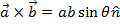, whereis a vector that is perpendicular to the other two. The units of angular momentum are kg∙m2/s.

angular momentum = (distance from the center of rotation) x (linear momentum)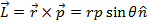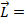angular momentum (kg∙m2/s)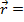length vector, directed from the center of rotation to the momentum point(meters)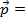linear momentum vector (kg∙m/s)

Angular Momentum Formula Questions:

1) A rubber ball attached to a string is being swung in a circle. The string is 0.500 m long, and the ball has a linear momentum of 4.00 kg∙m/s, which is always at a tangent to the circle the ball is spinning in. What is the magnitude of the ball’s angular momentum?

The length vector is in the direction of the string, extending from the center of rotation to the ball. The angle between the length vector and the linear momentum direction is 90°, and sin90° = 1. The angular momentum is: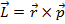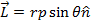The magnitude of the angular momentum was asked for, and so the direction of the angular momentum is not required:L = rp sinθL = rp(1)

L = (0.500 m)(4.00 kg∙m/s)

L = 2.00 kg∙m2/s

The magnitude of the angular momentum is 2.00 kg∙m2/s.

2) A lump of clay is sitting on the edge of a flat potter’s wheel. The wheel has a radius of 0.200 m, and the clay has a linear momentum of 3.60 kg∙m/s, which is always at a tangent to the circular edge of the potter’s wheel. What is the magnitude of the angular momentum of the clay?Answer:

The angle between the length vector and the linear momentum direction is 90°, and sin90° = 1. The angular momentum is:The magnitude of the angular momentum was asked for, and so the direction of the angular momentum is not required:L = rp sinθL = rp(1)

L = (0.200 m)(3.60 kg∙m/s)

L = 0.720 kg∙m2/s

The magnitude of the angular momentum is 0.720 kg∙m2/s.